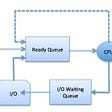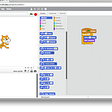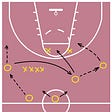# Python Algorithm: Pt. 10 Bellman Ford

`def get_edges(graph):n1 = []n2 = []weight = []for i in graph:for j in graph [i]:if graph[i][j] != 0:weight.append(graph[i][j])n1.append(i)n2.append(j)return n1, n2, weightdef bellman_ford(graph, start):n1, n2, weight = get_edges(graph)nodes = dict((i, INF) for i in graph)nodes[start] = 0for times in range(len(graph) - 1):cycle = 0for i in range (len(weight)):new_cost = nodes[n1[i]] + weight[i]if new_cost < nodes[n2[i]]:nodes[n2[i]] = new_costcycle = 1if cycle == 0:breakflag = 0for i in range(len(nodes)):if nodes[n1[i]] + weight[i] < nodes[n2[i]]:flag = 1breakif flag:return 'negative cycle'return nodesINF = 999graph = {'A':{'A':0, 'B':2, 'C':4},'B':{'B':0, 'C':7, 'E':6},'C':{'C':0, 'D':6, 'E':2},'D':{'D':0, 'E':8, 'G':4},'E':{'E':0, 'G':2},'G':{'G':0}}rtn = bellman_ford(graph, 'A')print(rtn)`

--

--

--

## More from Che Kai LIANG

Love podcasts or audiobooks? Learn on the go with our new app.

## Explaining how your computer decides on what to do next from a very high level.## From zero to the top, how I became a programmer.## CS 371p: Week 11## NET Core Avoiding large Controllers — using CQRS with MediatR.## The future of Ninja Gen## Substack Repost — OpenLampTech issue #29## Introducing of game play## Mobile App Development Using Python## Maintaining multiple versions of Python using Anaconda## Practice Making Simple Application Programs in Python for Beginners — Program 1: Tip Caculator